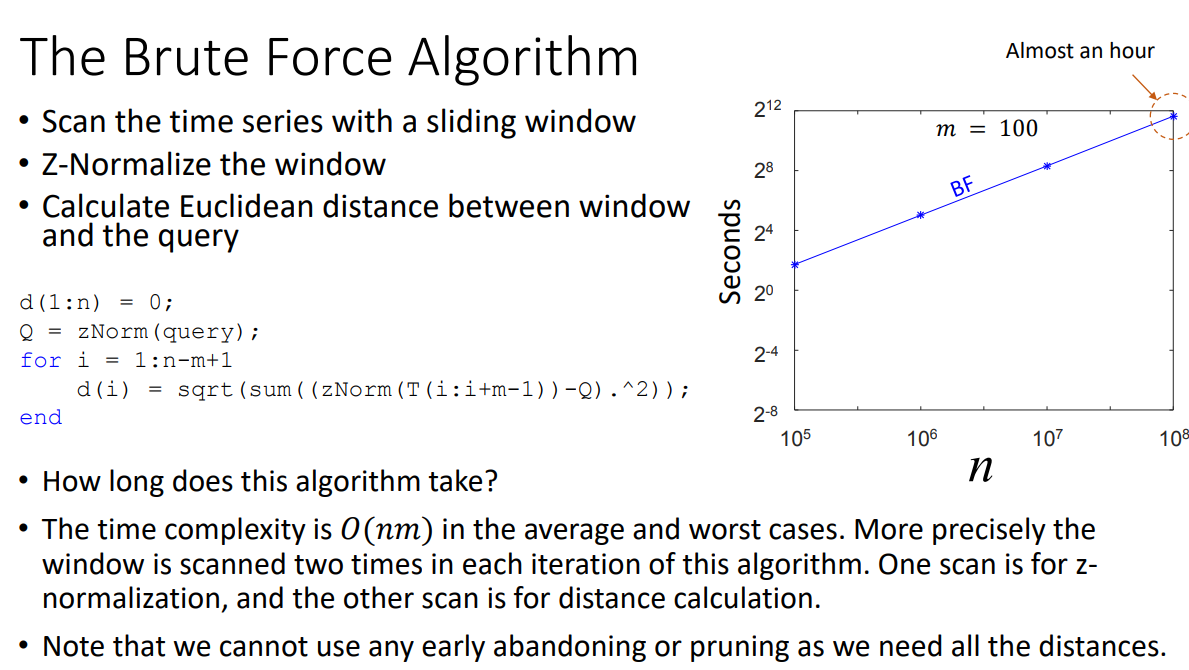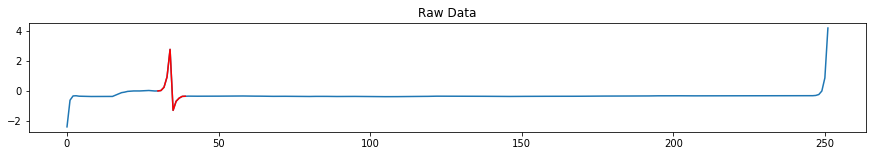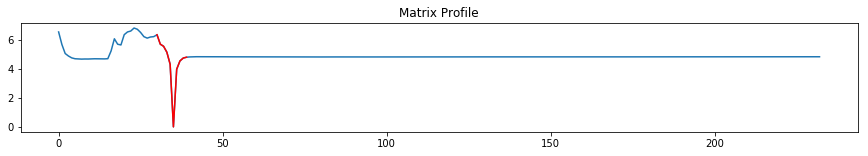Matrix Profile - Brute Force

This notebook is used to demonstrate the brute force algorithm for computing the matrix profile. This algorithm came from the slide deck:

https://www.cs.ucr.edu/~eamonn/Matrix_Profile_Tutorial_Part2.pdf

In :
from IPython.display import Image

In :
Image('images/bruteforce.png')

Out:In :
import numpy as np

In :
import warnings

In :
def znormalize(series):
series -= np.mean(series)
std = np.std(series)

if std == 0:
raise ValueError("The Standard Deviation cannot be zero")
else:
series /= std

return series

def brute_force_mp(series, window_size=None, query=None):
if isinstance(query, np.ndarray) and window_size:
warnings.warn('Query and window_size provided. Using query to determine window size.')

if isinstance(query, np.ndarray):
query = znormalize(query)
window_size = len(query)

#If length is odd, zero-pad time time series
if len(series) % 2 == 1:
profile = np.insert(profile, 0, 0)

#If length is odd, zero-pad query
if len(query) % 2 == 1:
query = np.insert(query, 0, 0)
window_size += 1

series_len = len(series)
shape = series_len - window_size + 1
profile = np.full(shape, np.inf)

for index in range(0, series_len - window_size + 1):
sub_sequence = series[index:index + window_size]
sub_sequence = znormalize(sub_sequence)
distance = np.sqrt(np.sum(np.power(sub_sequence - query, 2)))
profile[index] = distance

return profile


Data¶

The data consists of closing stock prices. I use the algorithm to identify which part of the data is closest to our subquery.

In :
series = np.loadtxt('stock_close.txt')
query = series[35:55]

mp = brute_force_mp(series, query=query)

In :
from matplotlib import pyplot as plt

%matplotlib inline

In :
plt.figure(figsize=(15,2))
plt.plot(series)
x = np.arange(30, 40)
y = series[x]
plt.plot(x, y, c='r')
plt.title('Raw Data')
plt.show()This plot shows the raw data and the subquery that is being searched for in red.

In :
plt.figure(figsize=(15,2))
plt.plot(mp)
x = np.arange(30, 40)
y = mp[x]
plt.plot(x, y, c='r')
plt.title('Matrix Profile')
plt.show()The matrix profile illustrates that the pattern was found hence the huge dip in the plot. This tells us that the distance between the subquery and that location of the series are very similar. In this case the subquery is identical. This pattern that is found is called a motif.